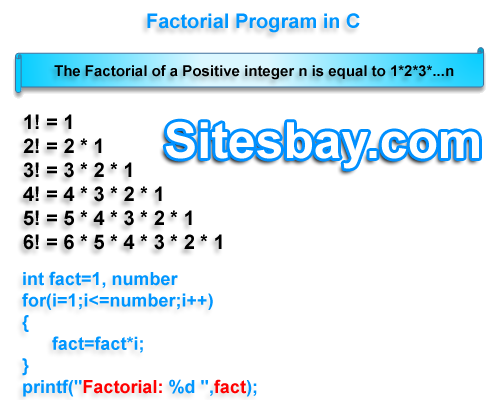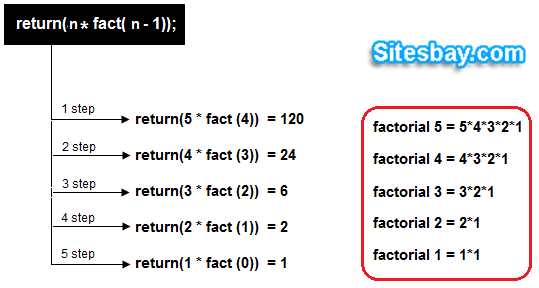# Factorial Program in C

## Find Factorial of Number Program in C

Factorial of any number is the product of an integer and all the integers below it.For example factorial of 4 is
4! = 4 * 3 * 2 * 1 = 24## Factorial of Number Program in C

```#include<stdio.h>
#include<conio.h>

void main()
{
int i, no, fact=1;
clrscr();
printf("Enter the any no. : ");
scanf("%d",&no);
for(i=1;i<=no;i++)
{
fact=fact*i;
}
printf("Factorial =%d ",fact);
getch();
}
```

## Output

```Enter the any no. : 4
Factorial = 24
```

## program factorial of number

```#include<stdio.h>
#include<conio.h>

void main()
{
long n, i, fact=1;
clrscr();
printf("Enter any number = ");
scanf("%ld",&n);
i=n;
if(n>=0)
{
do
{
fact=fact*i;
i--;
}
while(i>0);
printf("\nFactorial = %ld",fact);
}
else
{
printf("fact on -ve no is not possible");
}
getch();
}
```

## Output

```Enter the any no. : 5
Factorial = 120
```

### Factorial of number using recursion

The process in which a function calls itself directly or indirectly is called recursion and the corresponding function is called as recursive function. Here we write a factorial program using recurtion.## program factorial of number

```#include<stdio.h>
#include<conio.h>

void main()
{
int fact(int);
int f, n;
clrscr();
printf("ENTER ANY NUMBER = ");
scanf("%d",&n);
f=fact(n);
printf("\n\nFACTORIAL OF %d IS = %d",n,f);
getch();
}
int fact(int a)
{
if(a==1)
return(1);
else
{
return(a*fact(a-1));
}
}
```

## Output

```Enter the any no. : 6
Factorial = 720
```

WIFI CCTV Camera